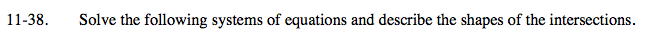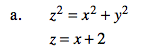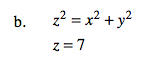### Home > A2C > Chapter Ch11 > Lesson 11.2.1 > Problem11-38

11-38.
1. Solve the following systems of equations and describe the shapes of the intersections. Homework Help ✎

1. z2 = x2 + y2
z = x + 2

2. z2 = x2 + y2
z = 7Substitute the second equation for z in the first, and simplify to find an equation of x and y.

What kind of graph is this?

$x =\frac{1}{4}y^2 -1$See part (a).